﻿ Parametric Study on Vessel Body Lines Modeling to Optimize Seakeeping Performance

### Parametric Study on Vessel Body Lines Modeling to Optimize Seakeeping PerformanceOPEN ACCESSPEER-REVIEWED

## Parametric Study on Vessel Body Lines Modeling to Optimize Seakeeping Performance

Mohsen Khosravi Babadi1, Hassan Ghassemi1,1Department of Ocean Engineering, AmirKabir University of Technology, Tehran, Iran

### Abstract

In this paper abody lines modeling algorithm for a corvettevesselis presented. In the algorithm seakeeping performance improved by variation in water-plane area coefficient (CWP) and consequently the vessel body lines modified. Fuzzy method used to body line modeling which the variation of CWP does not make change of the other geometric parameters (CP, CB, Cm, L and B). In this method, the impact of CWP changes on the rate of seakeeping parameter’s improvement has been studied and seakeeping performance index (SPI) defined as objective functions. Optimum value of seakeeping collective improvement obtained for the vessel body lines, using multi-objective genetic algorithms optimization (GA). Obviously, this method can be efficient in assuming that the other vessel geometrical coefficients are optimum.

### At a glance: Figures

12345
Prev Next

• Babadi, Mohsen Khosravi, and Hassan Ghassemi. "Parametric Study on Vessel Body Lines Modeling to Optimize Seakeeping Performance." Journal of Ocean Research 2.1 (2014): 5-10.
• Babadi, M. K. , & Ghassemi, H. (2014). Parametric Study on Vessel Body Lines Modeling to Optimize Seakeeping Performance. Journal of Ocean Research, 2(1), 5-10.
• Babadi, Mohsen Khosravi, and Hassan Ghassemi. "Parametric Study on Vessel Body Lines Modeling to Optimize Seakeeping Performance." Journal of Ocean Research 2, no. 1 (2014): 5-10.

 Import into BibTeX Import into EndNote Import into RefMan Import into RefWorks

### 1. Introduction

Seakeeping is the ability of a vessel to endure rough conditions at sea and navigate safely during long storms. The seakeeping performance is dynamic behavior of vessel in wind, waves and current. The performance can be expressed in terms of comfort, crew workability, damage to ship and cargo due to slamming and green water and in the end the earning capacity of the ship including the added resistance.

Each vessel has six degree of freedom include three linear and three angular motions. Determining of these operational motions, either analytical or non-analytical, can lead to the development of design conditions in preliminary steps of designing new vessels. Seakeeping performance depends on the hull form which is designed by naval architects to reach the optimum level.

Optimization in vessel seakeeping performance has long been of engineers' interest in naval architecture. Grigoropoulos presented on initial optimization of the main hull form for better seakeeping function. He defined the effective parameters in vessel seakeeping operation such as Cwp, LCF, CB, LCB, KB, and Cp and changed these parameters according to the original vessel hull form. His work extended the appropriate behavior of vessels in regular head-sea wave conditions by optimization algorithm and created optimized hull forms according to these parameters .

Kapsenbergtried to find the optimized hull form with respect to seakeeping capability through a program based on linear strip theory. This was implemented by investigation of three hull form parameters such as beam, draft and sectional area . Considering the constant speed of high-speed vessel in regular sinusoidal wave conditions carried out by Aranda et al. . Their experimental and analytical results had a good match in a vast domain of sea conditions. They studied heave, pitch, and roll dynamic motions in the encounter angles. Sarioz calculated 2-D damping and added mass coefficients using Frank Close-Fit method, and by his method he could investigate effects of some geometrical and hull form coefficient on seakeeping criteria .

Journee & Adegeest worked on the seakeeping related theories for ship design. Seakeeping theories for ships are introduced and considered as a practical method for calculating ship motions in waves . Other researchers like Alarrsinet al. , Davis and Holloway, and Sayli et al.carried out the problem solution of optimal hull forms and the others worked on the base of statistical analysis using a large amount of ship motion data related to various parameters in multi-linear models.

A comprehensive research on seakeeping behavior and hull form optimization investigated by Cepowski [9, 10] in Poland. He presented a method which makes it possible to determine optimum hull form of passenger car ferry with regard to selected sea-keeping qualities and added resistance in waves. His extension work did the modeling of a car passenger ferry ship design parameters with respect to these design criteria such as sea-keeping qualities and additional resistance in waves. He also researched to elaborate design guidelines which could make it possible to improve sea-keeping qualities of passenger-car ferries. The investigations for design guidelines were prepared in the form of regression functions as well as artificial neural networks on the basis of the results obtained from calculations with the use of numerical methods based on the theory of planar flow around a body.

Winyall  optimized a naval ship based on multi-objective optimization approach on seakeeping and resistance. Overall Measure of Effectiveness (OMOE) was used. In this method cost and risk considered beside use of relatively simple and traditional parametric hull form model and design variables (LBP, B, D, T, CP, CX, CRD) with performance based on parametric resistance algorithms. Khosrovari and Ghassemi used neural network to body line modeling where in CP changes indecently .

In this paper, a fuzzy model developed for the hull form generation. In this model body lines generated by CWP indecently variation which the variation of CWP do not make change of the other geometric parameters (CP, CB, Cm, L and B). Then seakeeping performance index (SPI) defined as objective functions which present dynamic behavior. Finally using multi-objective optimization, some values of CWP derived which optimize the seakeeping performance.

The geometric coefficient of study is water plan area coefficient of a vessel which few studies have been conducted. Here, a brief introduction to the coefficient using the standard approach presented. As Cm, the coefficient of water-plan fully covers the waterline. Waterline is one of the main elements of designing heavier vessels and it suggests the importance of vessel’s geometric coefficients. Waterline also has an important role in calculating the strength and stability of a vessel. CWP is calculated by using Equation (1):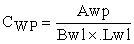(1)

Where Awp is longitudinal water-plane area and Lwl, Bwl are the width and length of the ship’s waterline, respectively, as shown in Figure 1.

### 2. Modeling fuzzy Changes in CWP

In this paper a model with fuzzy structure is presented for water-plan coefficient changes. As mentioned earlier, the variation of CWP does not make changes of the other geometric parameters. According to (Equation 1), the changes should be applied in the direction that no changes are made in the volume of a vessel. Soweneed the mathematical model of the changes that causes the exact changes in CWP and keep other geometric parameters constant with holding constant the volume. For this purpose, a fuzzy membership function, which is an extended bell membership function, is defined that it is making changes on the set of the body’s lines. So, if there are any other changes, it increases and decreases the same volume thus the total changes in the volume stay constant. The Bell function is described below, and its distribution shape is shown in Figure 2.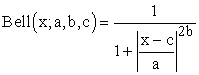(2)

As shown in the figure 2, the fuzzy function in the upper level make good changes in the middle of the body line and it returns the variant body lines to the original lines at both ends with instant cuts in two directions, so the bell applies as a coefficient of variation.

Now to restore the taken area of one side to the opposite direction the same function used in the interval (0, -1). So this function is multiplied by a sine function.

In this model, the fuzzy function coefficients are defined as follows: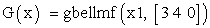(3)

a=3, b=4, c=0

Description of parameter effects on the function is given below:

Coefficient b: the coefficient is a positive number, and normally it is considered 4. This number is related to the upper part of the curve and gives that a bell-shaped curve and causes rise to the flat part of it.

The changes of this coefficient have a significant impact on the performance of the function. Figure 3 shows the changes in the crude bell membership function.

Coefficient a: with mathematical calculation on the lines of the body and modeling it, a coefficient equals to 1.44 times the length of each line of abody. Obviously, a coefficient of the vessel body lines will be different.

Coefficient c: This coefficient determines the center of the bell function that is considered equal to the half-length of each line.

The sine function of the fuzzy function is multiplied by Bell, is defined as follows: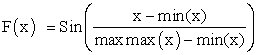(4)

Data set x is the length of along the body lines to a point where the fuzzy shift applies the same area to the other side.

The length of the new points is the function H (x). Coefficient L at the end of the function applies the different sizes of the changes manually.

This is the fuzzy function - neurological function obtained from Bell's model to apply changes to vessel model: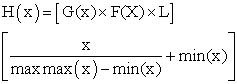(5)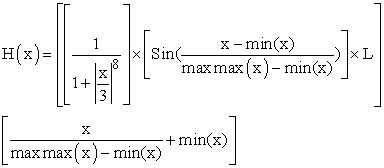(6)

Using Obtained membership function, the fuzzy inference system (FIS) is formed with a membership function H (x) and a simple inference functions (inference functions are set of simple if-then requirements).

Applying fuzzy relations based on customization done, applies the changes to any of the vessel body lines that are shown symbolically in Figure 4.

As it shown in figure 4, the output from the mentioned model does not changes the vessel volume and also it causes good changes on coefficient Cwp by changing the amount of AWP in Equation 1.

The integrity of this method in different vessel body lines is shown in Figure 5, which is selected of the chainless vessel.

2.1. The objective functions in the frequency domain

Using the base model, 10 variant models are generated for the coefficient CWP and seakeeping parameters RAO include roll, pitch, heave, added resistance and seasickness acceleration calculated in three heading angle. The dynamic behavior of a vessel is calculated for an irregular wave using strip theory method. The amounts of coefficient changes are very small so the results of calculations are very close together for different forms of the body. However, the differences in dynamic behavior are evident in some wave frequencies. The difference is mostly in the areas of maximum and minimum amplitude. This means that the purpose of optimization is reducing maximum amounts, thereby reducing the effective amounts of seakeeping parameters.

The 5 objective functions include roll, pitch, Heave, seasickness acceleration and added resistance in the waves heading angles of 120, 150 and 180 degrees, are plotted. And also due to the independent solving of roll equation in calculation, we will not have roll for 180 degree. Thus, many graphs obtained. Figure 6- Figure 9 presented as samples. Each diagram shows for CWP variation range of [-3%, +3%].

### 3. Multi-Objective Optimization With Seakeeping Purposes

For each generated geometric model and every heading angle, 5 RAO curve included: roll, pitch, Heave, added resistance and MSI will be produced based on the seasickness accelerations. Note that the optimization is not considered in a certain frequency; the significant value of every curve or parameter in frequency range will be calculated. For example, the coefficient of fineness (Cwp) with the percentage change of +3%, +2.5%, 2%, 1.5%0%, -1.5%, -2%, -2.5% and -3% and heading angle of 150 degrees can be considered 9 significant for each parameter. In order to achieve the objective functions from polynomial curve fitting is used contributing these 9 points. This objective function can be written to each coefficient (variable) at each heading angle. For determine the objective functions, each heading angle should be combined use of weights for any parameter(i.e., roll) achieved an objective function. In this case, the objective functions for the coefficient CWP is sorted in Table 1:

#### Table 1. weighting coefficients of Seakeeping parameters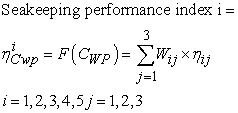(7)

Therefore, 5 objective functions can be defined for each coefficient. Furthermore, an additional objective function is added. The objective function represents the overall quality of the sea keeping. Depending on the vessel 5 functions may have different importance, for example, for the military's vessel, roll motion is more important than the added resistance. Thus, considering the weight coefficients, the seakeeping behavior is defined in Table 2.

#### Table 2. overall parameters of sea keeping objective functions

This objective functions (equation 8) can also be used when the importance of sea keeping parameters is different.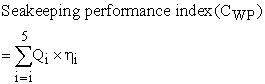(8)

The multiple objective functions are optimized using multi-objective genetic algorithm. In this paper the presented modelis applied on the vessel Body lines of a corvette vessel and the results improved. This improvement is shown in Figures 10-13, comparing the performance of the sea keeping model with optimized CWP and the original model of vessel.

Figure 13. Comparing the optimization and original model at vessel MSI parameters

### 4. Conclusions

In this study seakeeping performance of a corvette vessel considered and an improvement approach presented. First fuzzy model used to generate body line of a corvette vessel (from base model) so that CWP change to desired value and the variations don’t change other geometric coefficient. Then seakeeping RAO’s calculate by strip theory for generated models. Finally seakeeping performance indexes defined as objective function and optimized using multi objective genetic algorithm optimization.

The presented approach can be developed for other geometry coefficients.

Numerical results indicate that the performed modeling can be employed in a vessel hull design regarding the desired optimization conditions. Based our presented results, the following conclusions can be drawn:

1. fuzzy method has a good accuracy for vessel modeling and seakeeping survey.

2. Decreasing or increasing Cwp in the fore part of vessel can reduce deck wetness and increase slamming.

3. Decreasing or increasing Cwp in roll motion is totally related to the relative frequency and cannot be studied independent of it.

4. Decreasing Cwp results in a decrease in roll amplitude while increasing it leads to its increase.

### Acknowledgements

The authors would like to express their sincere appreciation for the respectable editor, who directed this paper so that it can be presented in the best standing. The authors would also like to thank there viewers for their helpful points and directions.

### References

  G. J. Grigoropoulos, “Hull Form Optimization for Hydrodynamic Performance,” Marine Technology, Vols. 41, No.4, pp. 167-182, (2004).In article  G. K. Kapsenberg, “Finding the Hullform for Given Seakeeping Characteristics,” MARIN, Wageningen, the Netherlands; IMAM, (2005).In article  J. Aranda, R. Munoz, S. D. Canto, J. M. Díaz and D. S. Bencomo, “An Analysis of Modes Identification Methods for High Speed Crafts,” Journal of Maritime Research, vol. 11, pp. 51-67, (2005).In article  E. Sarioz, “Inverse Design of Ship Hullforms for Seakeeping,” Ocean Engineering, vol. 36, pp. 1386-1395, (2009).In article CrossRef  J. Journée and L. Adegeest, “Theoretical Manual of Strip Theory Program “SEAWAY for Windows”,” Ship Hydromechanics Laboratory Delft University of Technology, Amarcon, (2003).In article  F. Alarrcin,U. Bugra Celebi,S. Ekinci and D. Ünsalan, “Neural Networks Based Analysis of Ship Roll Stabilization,”3rd International Conference on Maritime and Naval Science and Engineering, pp.217-220, (2010).In article  M. R. Davis and D. S. Holloway, “The Influence of Hullform on the Motions of High-speed Vessels in Head Seas,” Ocean Engineering, vol. 30, pp. 2091-2115, (2003).In article CrossRef  A. Sayli, A. Dursun A. and O. Ganiler, “Nonlinear meta- models for conceptual seakeeping design of fishing vessels,” Ocean Engineering, (2010).In article CrossRef  T. Cepowski, “Determination of optimum hull form for passenger car ferry with regard to its sea-keeping qualities and additional resistance in waves,” Polish Maritime Research, 2 (56) Vol 15, pp. 3-11, (2008).In article  T. Cepowski, “On the modeling of car passenger ferryship design parameters with respect to selected sea-keeping qualities and additional resistance in waves,” Polish Maritime Research, 3 (61) Vol 16, pp. 3-10, (2009).In article  D. Winyall, J. Edwards and A. Brown, “3D Hullform Modeling to Support Naval Ship Design Synthesis and Multi-Objective Optimization,” International Ship Design Conference (ISDC), Glasgow, Scotland, (2012).In article  M. K. Babadi, H. Ghassemi , “Effect of hull form coefficients on the vessel sea-keeping performance” Journal of Marine Science and Technology,Taiwan, Vol. 21, NO.5, 2013.In article